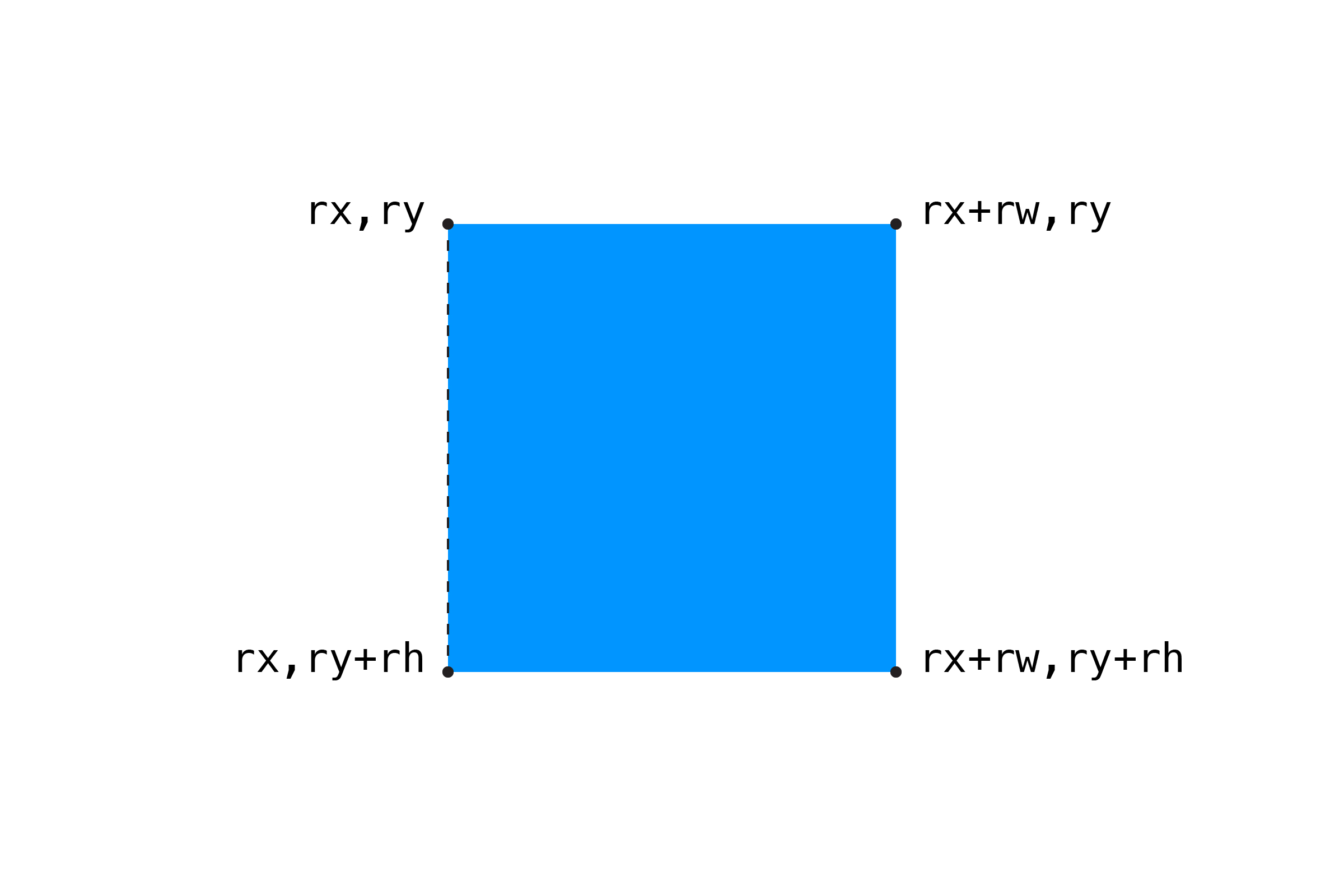[ your browser does not support the canvas tag ]

# POINT/RECTANGLE

Checking for collision with circular objects is fairly easy, since it is the same distance from the center to their edge in every direction. Rectangles require a bit more complex algorithm.

Let's say we have a square:

``````float rx = 10;  // x position
float ry = 10;  // y position
float rw = 30;  // width
float rh = 30;  // height
``````

To see if a point is inside the square, we have to test:

``````Is the X position of the point to the RIGHT of the LEFT EDGE?
Is the X position of the point to the LEFT of the RIGHT EDGE?
Is the Y position of the point BELOW the TOP EDGE?
Is the Y position of the point ABOVE the BOTTOM EDGE?
``````

If all of these are true, then the point is inside. Let's look at testing the left edge first. Since the default mode for the `rect()` command draws from the upper-left corner, the left edge is at `rx`:

``````if (px >= rx) {
// to the right of the left edge
}
``````

Pretty easy, but maybe not so intuitive. Here's a diagram showing the left edge of the rectangle:If we want to check the right edge, we need to get its X position, which is the left edge plus the width:

``````float rightEdge = rx + rw;
if (px <= rightEdge) {
// to the left of the right edge
}
``````

Here's the full if statement:

``````if (px >= rx &&         // right of the left edge AND
px <= rx + rw &&    // left of the right edge AND
py >= ry &&         // below the top AND
py <= ry + rh) {    // above the bottom
return true;
}
return false;
``````

If all the statements are true, then the point is inside the square. Note we can break our if statement into multiple lines, which makes it a little easier to read. This is personal preference, but we'll keep doing that here for the sake of clarity.

Here's a full example:

``````float px = 0;      // point position (move with mouse)
float py = 0;

float sx = 200;    // square position
float sy = 100;
float sw = 200;    // and dimensions
float sh = 200;

void setup() {
size(600,400);
noCursor();

strokeWeight(5);    // thicker stroke = easier to see
}

void draw() {
background(255);

// update point to mouse coordinates
px = mouseX;
py = mouseY;

// check for collision
// if hit, change rectangle color
boolean hit = pointRect(px,py, sx,sy,sw,sh);
if (hit) {
fill(255,150,0);
}
else {
fill(0,150,255);
}
noStroke();
rect(sx,sy, sw,sh);

// draw the point
stroke(0);
point(px,py);
}

// POINT/RECTANGLE
boolean pointRect(float px, float py, float rx, float ry, float rw, float rh) {

// is the point inside the rectangle's bounds?
if (px >= rx &&        // right of the left edge AND
px <= rx + rw &&   // left of the right edge AND
py >= ry &&        // below the top AND
py <= ry + rh) {   // above the bottom
return true;
}
return false;
}
``````

NEXT: Rectangle/Rectangle